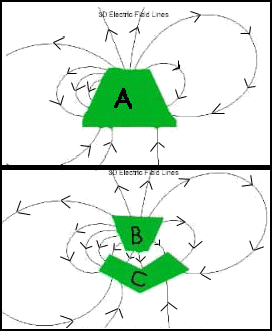Teaching Physics with the Physics Suite Edward F. Redish

Charge from field lines

The figures at the right show some representative electric field lines associated with some charges. Both pictures show the same charges and field lines, but they are masked in different ways by imaginary closed surfaces drawn for the purpose of hiding the charges from your view.

 A) From the field lines in the two pictures, which of the following statements is most likely to be true? a. There are no charges contained in A.  b. The charge contained in A is positive.  c. The charge contained in A is negative.  d. The total charge contained in A is zero.  e. None of the above can be true. B) From the field lines in the two pictures, which of the following statements is most likely to be true? a. There are no charges contained in B.  b. The charge contained in B is positive.  c. The charge contained in B is negative.  d. The total charge contained in B is zero.  e. None of the above. C) From the field lines in the two pictures, which of the following statements is most likely to be true? a The charge contained in C is positive and greater in magnitude than the charge in B.  b. The charge contained in C is positive and smaller in magnitude than the charge in B.  c. The charge contained in C is negative and greater in magnitude than the charge in B.  d. The total charge contained in C is negative and smaller in magnitude than the charge in B.  e. None of the above.D) From the information in the two pictures, what can you say about the electrostatic potential at a point on the surface of box B compared to the electrostatic potential on the surface of box C?

a. The potential of any point on the surface of B is higher than the potential of any point on the surface of C.
b. The potential of any point on the surface of C is higher than the potential of any point on the surface of B.
c. The potential on the surface B and C are the same.
d. Some of the points on B will be at a higher potential than some of the points on C, but not all of them.
e. Nothing can be determined about the relative potentials.

Not finding what you wanted? Check the Site Map for more information.